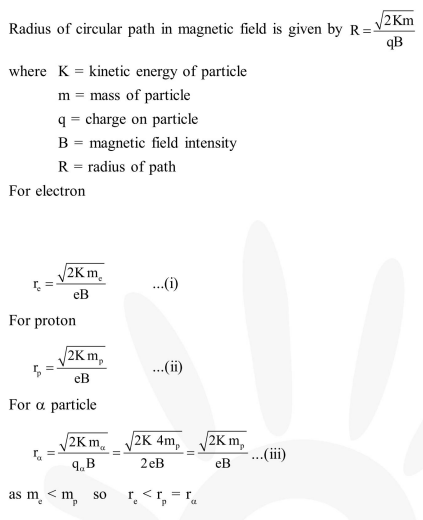# An electron, a proton and an alpha particle having`
Question:

An electron, a proton and an alpha particle having the same kinetic energy are moving in circular orbits of radii $\mathrm{r}_{\mathrm{e}}, \mathrm{r}_{\mathrm{p}}, \mathrm{r}_{\alpha}$ respectively in a uniform magnetic field $\mathrm{B}$. The relation between $\mathrm{r}_{\mathrm{e}}, \mathrm{r}_{\mathrm{p}}, \mathrm{r}_{\alpha}$ is:-

1. $\mathrm{r}_{\mathrm{e}}<\mathrm{r}_{\mathrm{p}}=\mathrm{r}_{\alpha}$

2. $\mathrm{r}_{\mathrm{e}}<\mathrm{r}_{\mathrm{p}}<\mathrm{r}_{\alpha}$

3. $\mathrm{r}_{\mathrm{e}}<\mathrm{r}_{\alpha}<\mathrm{r}_{\mathrm{p}}$

4. $\mathrm{r}_{\mathrm{e}}>\mathrm{r}_{\mathrm{p}}=\mathrm{r}_{\alpha}$

Correct Option: 1

Solution: